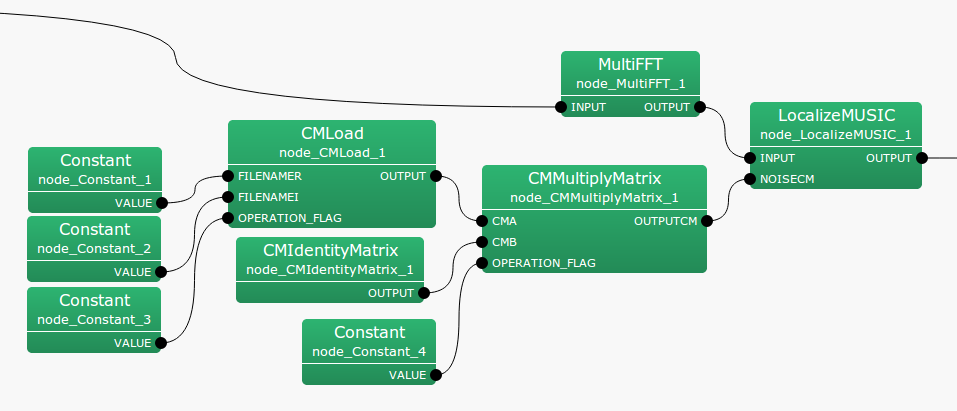6.2.10 CMMultiplyMatrix

6.2.10.1 Module Overview

Multiply each frequency bin of the two sound source correlation matrices.

None.

6.2.10.3 Usage

In what case is the node used?

The calculation node of the sound source correlation matrix is the same as the one from CMMakerFromFFT , CMMakerFromFFTwithFlag , and has the function of multiplying the correlation matrix of each frequency bin.

Typical Examples

Figure. 6.26 shows the usage example of CMMultiplyMatrix  node.

CMA input terminal is connected to the correlation matrix calculated from CMMakerFromFFT , CMMakerFromFFTwithFlag , etc. (Type is Matrix<complex<float> >  type, but to handle a correlation matrix, convert the three-dimensional complex array to a two-dimensional complex matrix and then output). The CMB input terminal, like CMA, connects to the same correlation matrix. At the time of multiplication, CMA $*$ CMB is calculated for each frequency bin. OPERATION_FLAG is an int  type or bool  type input, specifying when the correlation matrix is calculated.Figure 6.26: Network Example using CMMultiplyMatrix

6.2.10.4 I/O and property setting of the node

Table 6.27: Parameter list of CMMultiplyMatrix
 Parameter Type Default Unit Description FIRST_FRAME_EXECUTION false Selection of the first frame execution ENABLE_DEBUG false ON/OFF of debugging information output

Input

CMA

: Matrix<complex<float> >  type. A correlation matrix for each frequency bin. The $M$-th order complex square array correlation matrix inputs $NFFT/2 + 1$ items. Matrix<complex<float> >  contains rows corresponding to frequencies ($NFFT/2 + 1$ rows) and the columns contains the complex correlation matrix ($M * M$ columns across).

CMB

: Matrix<complex<float> >  type. Same as CMA.

OPERATION_FLAG

: int  type or bool  type. Only when this input terminal is 1 or true, calculation of the correlation matrix is performed.

Output

OUTPUTCM

: Matrix<complex<float> >  type. Correlation matrix equivalent to CMA $*$ CMB, after multiplication, is output.

Parameter

FIRST_FRAME_EXECUTION

: bool  type. The default value is false. When true, OPERATION_FLAG is always 0. Even if false, perform only the calculation of first frame.

ENABLE_DEBUG

: bool  type. Default value is false. When true, output the frame number calculated to the standard output, during correlation matrix multiplication.

6.2.10.5 Module Description

Performs the multiplication of two correlation matrices for each frequency bin. The correlation matrix is a complex three-dimensional array of size $k \times M \times M$ and the multiplication $k$ times is performed as follows. Here, $k$ is the number of frequency bins ($k = NFFT/2 + 1$) and $M$ is a number of channels in the input signal.

OUTPUTCM = zero_matrix(k,M,M)

calculate{

IF OPERATION_FLAG
FOR i = 1 to k

OUTPUTCM[i] = CMA[i] * CMB[i]

ENDFOR
ENDIF

}

The matrix that is output from the OUTPUTCM terminal is initialized as a zero matrix, and maintains the final operational result from this point onward.